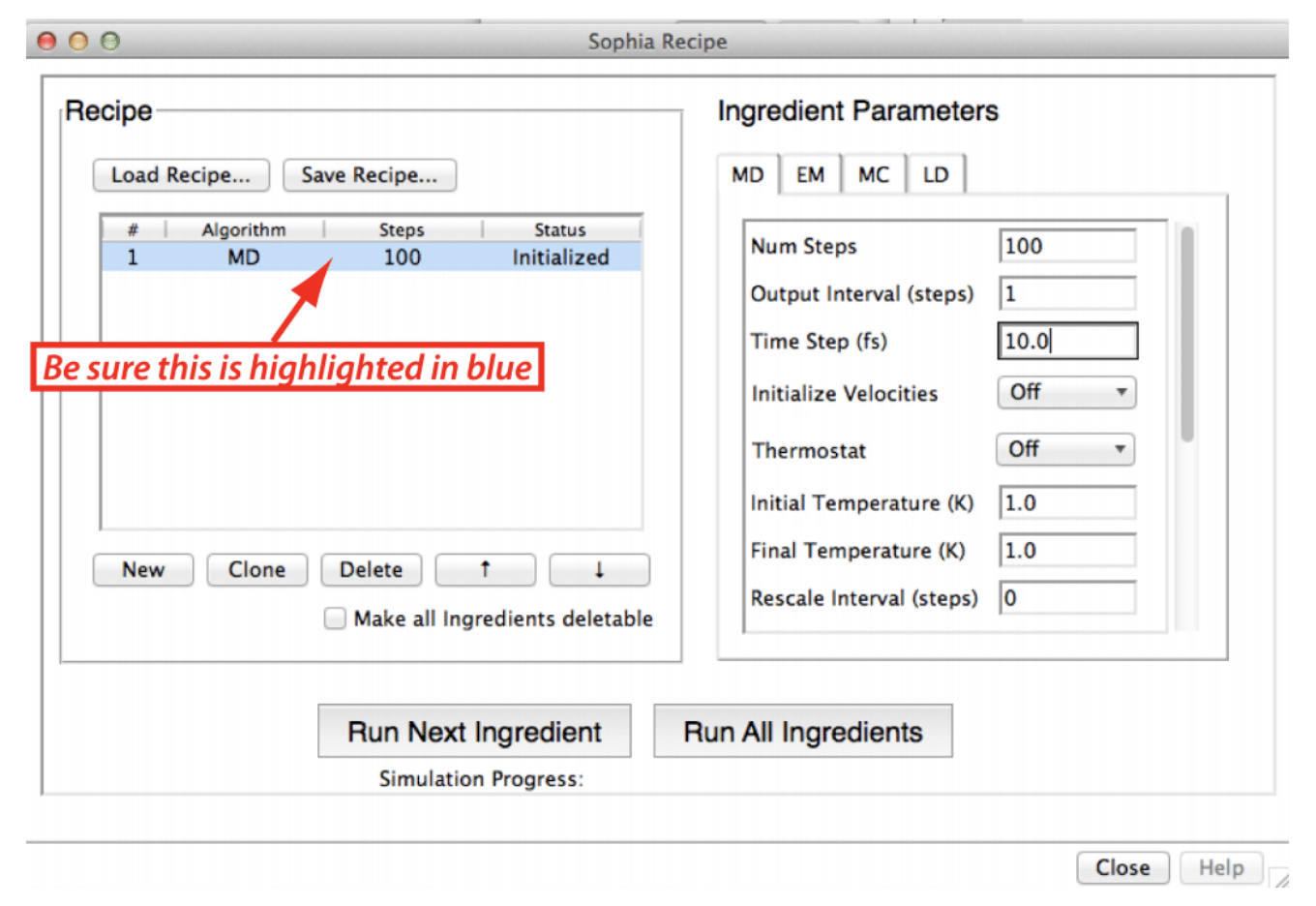# Tutorial 3 Setting the Time Step

## 3.1 Introduction

In this tutorial, you will carry out a series of MD simulations on the harmonic oscillator, varying the size of the time step and monitoring the accuracy, to determine the maximum acceptable step size.The molecular dynamics algorithm represents the numerical integration of Newton’s equations of motion. This requires that time be represented by discrete intervals, each of length $$\Delta t$$. Obviously, the smaller $$\Delta t$$, the more accurately will the MD simulation reproduce the system’s actual behavior. But there are other demands on the computational resources that compete with the desire for accuracy – the desire to simulate large systems, and the desire to cover events that occur only over long time scales. So, we want $$\Delta t$$ to be as large as possible. The question then becomes, how large can $$\Delta t$$ be before the accuracy of the simulation is seriously degraded? In short: We want the time step to be as large as possible, but not too large.

## 3.2 Running the simulation

1. Open Chimera and launch Sophia. In the Sophia Main window, click Load Simulation and load the file harm_osc1.sophia, which you created in Tutorial 1. In the Sophia Universe window, click Reset Universe.

2. In the Sophia Recipe window, click Load Recipe and choose harmonic_oscillator_recipe.json (the same as in Tutorial 1)

3. Click on the recipe in the left half of the window and be sure it’s highlighted in blue as in the figure. Then, on the right side of the Sophia Recipe window, edit Ingredient Parameters to be:

• Num steps: 100
• Output interval (steps): 1
• Time step (fs): 10.0
• (other settings unchanged)1. Click Run Next Ingredient

## 3.3 Analysis and follow-up simulations

1. Since this simulation took 100 steps x 10 fs/step, it covers 1 ps, the same interval covered in the first harmonic oscillator tutorial, which had a time step of 1 fs. Compare the behavior of the system ($$E_{pot}$$; $$E_{kin}$$; $$E_{tot}$$; bond length) in the two simulations ($$\Delta t$$ = 1 fs vs. $$\Delta t$$ = 10 fs). Is the 10 fs time step acceptable?

2. To examine the behavior with a longer time step, reset the universe (click Reset Universe in the Sophia Universe window) and repeat the steps above, except with $$\Delta t$$ = 100 fs. Is the 100 fs time step acceptable?

3. If necessary, repeat the above steps with still longer time steps, until $$\Delta t$$ is so large that you decide it is unacceptable. What is your criterion for “unacceptability”?

4. Experiment with intermediate values of $$\Delta t$$. Is there a sharp break between acceptable and unacceptable values, or does the quality of the simulation deteriorate gradually as $$\Delta t$$ gets larger and larger?